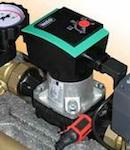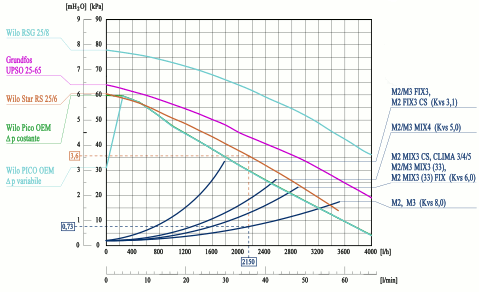ItalianEnglish

### Contact now

invalid value
invalid value
invalid value
invalid value
Invalid value, do not insert the (country code +39)
invalid value
invalid value
invalid value
invalid value
invalid value
invalid value### Circulators for heating water without problems

The choice of the most suitable pump is fundamental to ensure a plant flow such that develops the power established at the design stage and for avoid problems in operation.

Knowing this fact, and considering the Dt Dt between flow and return, we can obtain the flow rate in kg / h.

It 'also a fact that the type of module is used, we already know a priori as chosen according to the type of plant to be realized.

EXAMPLE: For a plant that uses a group led M2 and that requires a power P = 50 kW a Dt Dt = K 20 calculate the flow rate:

##### (50 kW X 860): 20K = 2150 kg / h

We now need to quantify the pressure drop of our system, so as to choose a circulator that is not undersized.

As for the form, we can know the load losses on the following diagram the curve for the desired pattern.

We find in our case that the group of direct pumping M2 at a flow rate of 2150 kg / h corresponds to a pressure drop of 0,75 m of water column.It is to be addition la overall pressure drop plant (pipes, fittings, radiant elements, etc..) that must be supplied to a given by the designer.

From the graph, the circulator Star RS 25 / 6 for a flow rate of 2150 kg / h has a prevalence of 3,6 m.: considered that, the module absorbs 0,73 m, remain 2,87 m (as 3,6 - 0,73 2,87 = m) of water column available to compensate for the load losses plant.

If this data is sufficient, we can use the Star RS 25/6, otherwise the pump model with higher head must be used.

NOTE: to the desired flow rate, if necessary, it is possible to calculate mathematical procedure with the pressure drop caused by the presence of a hydraulic device, when you know the Kvs; therefore, with good approximation, assuming the standard temperature equal to 20 ° C and neglecting the effects due to the viscosity of the fluid, is in fact:

##### Kvs = Q: √ h

when the scope Q is expressed in m3 / h e h, The pressure difference at the ends of the device (pressure drop), in bar.

Reversing the above formula is obtained:

##### h = (Q: Kvs)2

essendo 1 bar corresponding to approximately 10,198 mH2O, The pressure drop is therefore equal to 0,73 mH2OAs shown in the diagram.